# Cake Eating II: Numerical Methods¶

## Contents¶

In addition to what’s in Anaconda, this lecture will require the following library:

In :
!pip install interpolation


## Overview¶

In this lecture we continue the study of the cake eating problem.

The aim of this lecture is to solve the problem using numerical methods.

At first this might appear unnecessary, since we already obtained the optimal policy analytically.

However, the cake eating problem is too simple to be useful without modifications, and once we start modifying the problem, numerical methods become essential.

Hence it makes sense to introduce numerical methods now, and test them on this simple problem.

Since we know the analytical solution, this will allow us to assess the accuracy of alternative numerical methods.

We will use the following imports:

In :
import numpy as np
import matplotlib.pyplot as plt
%matplotlib inline

from interpolation import interp
from scipy.optimize import minimize_scalar, bisect


## Reviewing the Model¶

You might like to review the details before we start.

Recall in particular that the Bellman equation is

$$v(x) = \max_{0\leq c \leq x} \{u(c) + \beta v(x-c)\} \quad \text{for all } x \geq 0. \tag{1}$$

where $u$ is the CRRA utility function.

The analytical solutions for the value function and optimal policy were found to be as follows.

In :
def c_star(x, β, γ):

return (1 - β ** (1/γ)) * x

def v_star(x, β, γ):

return (1 - β**(1 / γ))**(-γ) * (x**(1-γ) / (1-γ))


Our first aim is to obtain these analytical solutions numerically.

## Value Function Iteration¶

The first approach we will take is value function iteration.

This is a form of successive approximation, and was discussed in our lecture on job search.

The basic idea is:

1. Take an arbitary intial guess of $v$.
2. Obtain an update $w$ defined by
$$w(x) = \max_{0\leq c \leq x} \{u(c) + \beta v(x-c)\}$$
3. Stop if $w$ is approximately equal to $v$, otherwise set $v=w$ and go back to step 2.

Let’s write this a bit more mathematically.

### The Bellman Operator¶

We introduce the Bellman operator $T$ that takes a function v as an argument and returns a new function $Tv$ defined by

$$Tv(x) = \max_{0 \leq c \leq x} \{u(c) + \beta v(x - c)\}$$

From $v$ we get $Tv$, and applying $T$ to this yields $T^2 v := T (Tv)$ and so on.

This is called iterating with the Bellman operator from initial guess $v$.

As we discuss in more detail in later lectures, one can use Banach’s contraction mapping theorem to prove that the sequence of functions $T^n v$ converges to the solution to the Bellman equation.

### Fitted Value Function Iteration¶

Both consumption $c$ and the state variable $x$ are continuous.

This causes complications when it comes to numerical work.

For example, we need to store each function $T^n v$ in order to compute the next iterate $T^{n+1} v$.

But this means we have to store $T^n v(x)$ at infinitely many $x$, which is, in general, impossible.

To circumvent this issue we will use fitted value function iteration, as discussed previously in one of the lectures on job search.

The process looks like this:

1. Begin with an array of values $\{ v_0, \ldots, v_I \}$ representing the values of some initial function $v$ on the grid points $\{ x_0, \ldots, x_I \}$.
2. Build a function $\hat v$ on the state space $\mathbb R_+$ by linear interpolation, based on these data points.
3. Obtain and record the value $T \hat v(x_i)$ on each grid point $x_i$ by repeatedly solving the maximization problem in the Bellman equation.
4. Unless some stopping condition is satisfied, set $\{ v_0, \ldots, v_I \} = \{ T \hat v(x_0), \ldots, T \hat v(x_I) \}$ and go to step 2.

In step 2 we’ll use continuous piecewise linear interpolation.

### Implementation¶

The maximize function below is a small helper function that converts a SciPy minimization routine into a maximization routine.

In :
def maximize(g, a, b, args):
"""
Maximize the function g over the interval [a, b].

We use the fact that the maximizer of g on any interval is
also the minimizer of -g.  The tuple args collects any extra
arguments to g.

Returns the maximal value and the maximizer.
"""

objective = lambda x: -g(x, *args)
result = minimize_scalar(objective, bounds=(a, b), method='bounded')
maximizer, maximum = result.x, -result.fun
return maximizer, maximum


We’ll store the parameters $\beta$ and $\gamma$ in a class called CakeEating.

The same class will also provide a method called state_action_value that returns the value of a consumption choice given a particular state and guess of $v$.

In :
class CakeEating:

def __init__(self,
β=0.96,           # discount factor
γ=1.5,            # degree of relative risk aversion
x_grid_min=1e-3,  # exclude zero for numerical stability
x_grid_max=2.5,   # size of cake
x_grid_size=120):

self.β, self.γ = β, γ

# Set up grid
self.x_grid = np.linspace(x_grid_min, x_grid_max, x_grid_size)

# Utility function
def u(self, c):

γ = self.γ

if γ == 1:
return np.log(c)
else:
return (c ** (1 - γ)) / (1 - γ)

# first derivative of utility function
def u_prime(self, c):

return c ** (-self.γ)

def state_action_value(self, c, x, v_array):
"""
Right hand side of the Bellman equation given x and c.
"""

u, β = self.u, self.β
v = lambda x: interp(self.x_grid, v_array, x)

return u(c) + β * v(x - c)


We now define the Bellman operation:

In :
def T(v, ce):
"""
The Bellman operator.  Updates the guess of the value function.

* ce is an instance of CakeEating
* v is an array representing a guess of the value function

"""
v_new = np.empty_like(v)

for i, x in enumerate(ce.x_grid):
# Maximize RHS of Bellman equation at state x
v_new[i] = maximize(ce.state_action_value, 1e-10, x, (x, v))

return v_new


After defining the Bellman operator, we are ready to solve the model.

Let’s start by creating a CakeEating instance using the default parameterization.

In :
ce = CakeEating()


Now let’s see the iteration of the value function in action.

We start from guess $v$ given by $v(x) = u(x)$ for every $x$ grid point.

In :
x_grid = ce.x_grid
v = ce.u(x_grid)       # Initial guess
n = 12                 # Number of iterations

fig, ax = plt.subplots()

ax.plot(x_grid, v, color=plt.cm.jet(0),
lw=2, alpha=0.6, label='Initial guess')

for i in range(n):
v = T(v, ce)  # Apply the Bellman operator
ax.plot(x_grid, v, color=plt.cm.jet(i / n), lw=2, alpha=0.6)

ax.legend()
ax.set_ylabel('value', fontsize=12)
ax.set_xlabel('cake size $x$', fontsize=12)
ax.set_title('Value function iterations')

plt.show()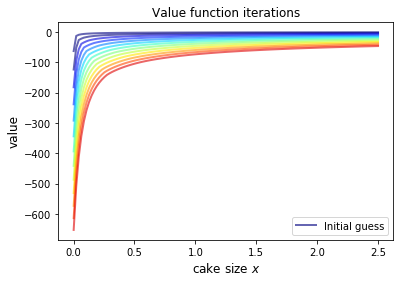To do this more systematically, we introduce a wrapper function called compute_value_function that iterates until some convergence conditions are satisfied.

In :
def compute_value_function(ce,
tol=1e-4,
max_iter=1000,
verbose=True,
print_skip=25):

# Set up loop
v = np.zeros(len(ce.x_grid)) # Initial guess
i = 0
error = tol + 1

while i < max_iter and error > tol:
v_new = T(v, ce)

error = np.max(np.abs(v - v_new))
i += 1

if verbose and i % print_skip == 0:
print(f"Error at iteration {i} is {error}.")

v = v_new

if i == max_iter:
print("Failed to converge!")

if verbose and i < max_iter:
print(f"\nConverged in {i} iterations.")

return v_new


Now let’s call it, noting that it takes a little while to run.

In :
v = compute_value_function(ce)

Error at iteration 25 is 23.8003755134813.
Error at iteration 50 is 8.577577195046615.
Error at iteration 75 is 3.091330659691039.
Error at iteration 100 is 1.1141054204751981.
Error at iteration 125 is 0.4015199357729671.
Error at iteration 150 is 0.14470646660561215.
Error at iteration 175 is 0.052151735472762084.
Error at iteration 200 is 0.018795314242879613.
Error at iteration 225 is 0.006773769545588948.
Error at iteration 250 is 0.0024412443051460286.
Error at iteration 275 is 0.000879816432870939.
Error at iteration 300 is 0.00031708295398402697.
Error at iteration 325 is 0.00011427565573285392.

Converged in 329 iterations.


Now we can plot and see what the converged value function looks like.

In :
fig, ax = plt.subplots()

ax.plot(x_grid, v, label='Approximate value function')
ax.set_ylabel('$V(x)$', fontsize=12)
ax.set_xlabel('$x$', fontsize=12)
ax.set_title('Value function')
ax.legend()
plt.show()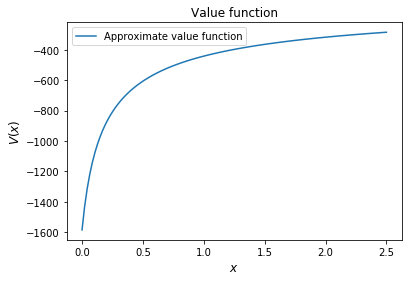Next let’s compare it to the analytical solution.

In :
v_analytical = v_star(ce.x_grid, ce.β, ce.γ)

In :
fig, ax = plt.subplots()

ax.plot(x_grid, v_analytical, label='analytical solution')
ax.plot(x_grid, v, label='numerical solution')
ax.set_ylabel('$V(x)$', fontsize=12)
ax.set_xlabel('$x$', fontsize=12)
ax.legend()
ax.set_title('Comparison between analytical and numerical value functions')
plt.show()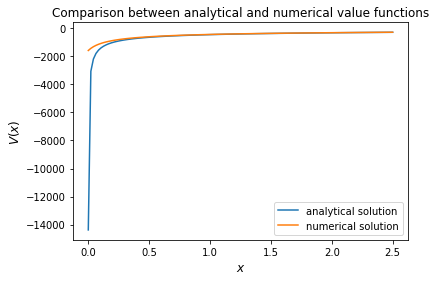The quality of approximation is reasonably good for large $x$, but less so near the lower boundary.

The reason is that the utility function and hence value function is very steep near the lower boundary, and hence hard to approximate.

### Policy Function¶

Let’s see how this plays out in terms of computing the optimal policy.

In the first lecture on cake eating, the optimal consumption policy was shown to be

$$\sigma^*(x) = \left(1-\beta^{1/\gamma} \right) x$$

Let’s see if our numerical results lead to something similar.

Our numerical strategy will be to compute

$$\sigma(x) = \arg \max_{0 \leq c \leq x} \{u(c) + \beta v(x - c)\}$$

on a grid of $x$ points and then interpolate.

For $v$ we will use the approximation of the value function we obtained above.

Here’s the function:

In :
def σ(ce, v):
"""
The optimal policy function. Given the value function,
it finds optimal consumption in each state.

* ce is an instance of CakeEating
* v is a value function array

"""
c = np.empty_like(v)

for i in range(len(ce.x_grid)):
x = ce.x_grid[i]
# Maximize RHS of Bellman equation at state x
c[i] = maximize(ce.state_action_value, 1e-10, x, (x, v))

return c


Now let’s pass the approximate value function and compute optimal consumption:

In :
c = σ(ce, v)


Let’s plot this next to the true analytical solution

In :
c_analytical = c_star(ce.x_grid, ce.β, ce.γ)

fig, ax = plt.subplots()

ax.plot(ce.x_grid, c_analytical, label='analytical')
ax.plot(ce.x_grid, c, label='numerical')
ax.set_ylabel(r'$\sigma(x)$')
ax.set_xlabel('$x$')
ax.legend()

plt.show()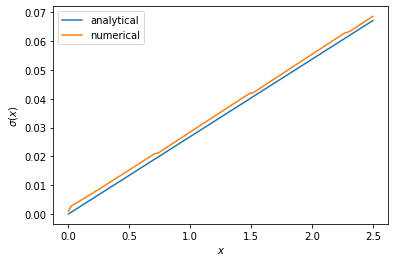The fit is reasonable but not perfect.

We can improve it by increasing the grid size or reducing the error tolerance in the value function iteration routine.

However, both changes will lead to a longer compute time.

Another possibility is to use an alternative algorithm, which offers the possibility of faster compute time and, at the same time, more accuracy.

We explore this next.

## Time Iteration¶

Now let’s look at a different strategy to compute the optimal policy.

Recall that the optimal policy satisfies the Euler equation

$$u' (\sigma(x)) = \beta u' ( \sigma(x - \sigma(x))) \quad \text{for all } x > 0 \tag{2}$$

Computationally, we can start with any initial guess of $\sigma_0$ and now choose $c$ to solve

$$u^{\prime}( c ) = \beta u^{\prime} (\sigma_0(x - c))$$

Choosing $c$ to satisfy this equation at all $x > 0$ produces a function of $x$.

Call this new function $\sigma_1$, treat it as the new guess and repeat.

This is called time iteration.

As with value function iteration, we can view the update step as action of an operator, this time denoted by $K$.

• In particular, $K\sigma$ is the policy updated from $\sigma$ using the procedure just described.
• We will use this terminology in the exercises below.

The main advantage of time iteration relative to value function iteration is that it operates in policy space rather than value function space.

This is helpful because the policy function has less curvature, and hence is easier to approximate.

In the exercises you are asked to implement time iteration and compare it to value function iteration.

You should find that the method is faster and more accurate.

This is due to

1. the curvature issue mentioned just above and
2. the fact that we are using more information — in this case, the first order conditions.

## Exercises¶

### Exercise 1¶

Try the following modification of the problem.

Instead of the cake size changing according to $x_{t+1} = x_t - c_t$, let it change according to

$$x_{t+1} = (x_t - c_t)^{\alpha}$$

where $\alpha$ is a parameter satisfying $0 < \alpha < 1$.

(We will see this kind of update rule when we study optimal growth models.)

Make the required changes to value function iteration code and plot the value and policy functions.

Try to reuse as much code as possible.

### Exercise 2¶

Implement time iteration, returning to the original case (i.e., dropping the modification in the exercise above).

## Solutions¶

### Exercise 1¶

We need to create a class to hold our primitives and return the right hand side of the Bellman equation.

We will use inheritance to maximize code reuse.

In :
class OptimalGrowth(CakeEating):
"""
A subclass of CakeEating that adds the parameter α and overrides
the state_action_value method.
"""

def __init__(self,
β=0.96,           # discount factor
γ=1.5,            # degree of relative risk aversion
α=0.4,            # productivity parameter
x_grid_min=1e-3,  # exclude zero for numerical stability
x_grid_max=2.5,   # size of cake
x_grid_size=120):

self.α = α
CakeEating.__init__(self, β, γ, x_grid_min, x_grid_max, x_grid_size)

def state_action_value(self, c, x, v_array):
"""
Right hand side of the Bellman equation given x and c.
"""

u, β, α = self.u, self.β, self.α
v = lambda x: interp(self.x_grid, v_array, x)

return u(c) + β * v((x - c)**α)

In :
og = OptimalGrowth()


Here’s the computed value function.

In :
v = compute_value_function(og, verbose=False)

fig, ax = plt.subplots()

ax.plot(x_grid, v, lw=2, alpha=0.6)
ax.set_ylabel('value', fontsize=12)
ax.set_xlabel('state $x$', fontsize=12)

plt.show()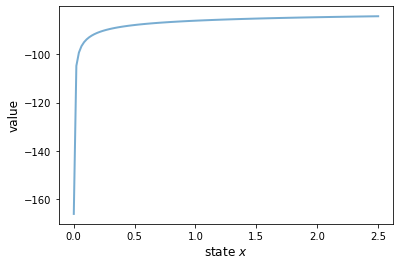Here’s the computed policy, combined with the solution we derived above for the standard cake eating case $\alpha=1$.

In :
c_new = σ(og, v)

fig, ax = plt.subplots()

ax.plot(ce.x_grid, c_analytical, label=r'$\alpha=1$ solution')
ax.plot(ce.x_grid, c_new, label=fr'$\alpha={og.α}$ solution')

ax.set_ylabel('consumption', fontsize=12)
ax.set_xlabel('$x$', fontsize=12)

ax.legend(fontsize=12)

plt.show()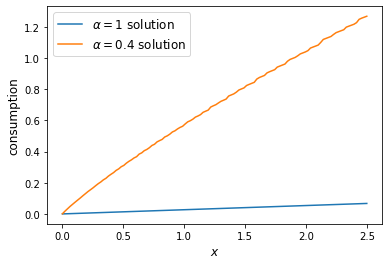Consumption is higher when $\alpha < 1$ because, at least for large $x$, the return to savings is lower.

### Exercise 2¶

Here’s one way to implement time iteration.

In :
def K(σ_array, ce):
"""
The policy function operator. Given the policy function,
it updates the optimal consumption using Euler equation.

* σ_array is an array of policy function values on the grid
* ce is an instance of CakeEating

"""

u_prime, β, x_grid = ce.u_prime, ce.β, ce.x_grid
σ_new = np.empty_like(σ_array)

σ = lambda x: interp(x_grid, σ_array, x)

def euler_diff(c, x):
return u_prime(c) - β * u_prime(σ(x - c))

for i, x in enumerate(x_grid):

# handle small x separately --- helps numerical stability
if x < 1e-12:
σ_new[i] = 0.0

# handle other x
else:
σ_new[i] = bisect(euler_diff, 1e-10, x - 1e-10, x)

return σ_new

In :
def iterate_euler_equation(ce,
max_iter=500,
tol=1e-5,
verbose=True,
print_skip=25):

x_grid = ce.x_grid

σ = np.copy(x_grid)        # initial guess

i = 0
error = tol + 1
while i < max_iter and error > tol:

σ_new = K(σ, ce)

error = np.max(np.abs(σ_new - σ))
i += 1

if verbose and i % print_skip == 0:
print(f"Error at iteration {i} is {error}.")

σ = σ_new

if i == max_iter:
print("Failed to converge!")

if verbose and i < max_iter:
print(f"\nConverged in {i} iterations.")

return σ

In :
ce = CakeEating(x_grid_min=0.0)
c_euler = iterate_euler_equation(ce)

Error at iteration 25 is 0.0036456675931543225.
Error at iteration 50 is 0.0008283185047067848.
Error at iteration 75 is 0.00030791132300957147.
Error at iteration 100 is 0.00013555502390599772.
Error at iteration 125 is 6.417740905302616e-05.
Error at iteration 150 is 3.1438019047758115e-05.
Error at iteration 175 is 1.5658492883291464e-05.

Converged in 192 iterations.

In :
fig, ax = plt.subplots()

ax.plot(ce.x_grid, c_analytical, label='analytical solution')
ax.plot(ce.x_grid, c_euler, label='time iteration solution')

ax.set_ylabel('consumption')
ax.set_xlabel('$x$')
ax.legend(fontsize=12)

plt.show()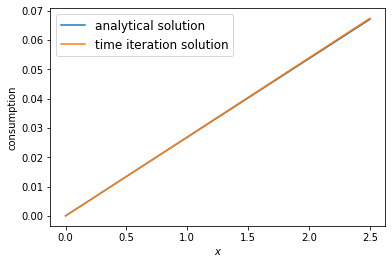• Share page